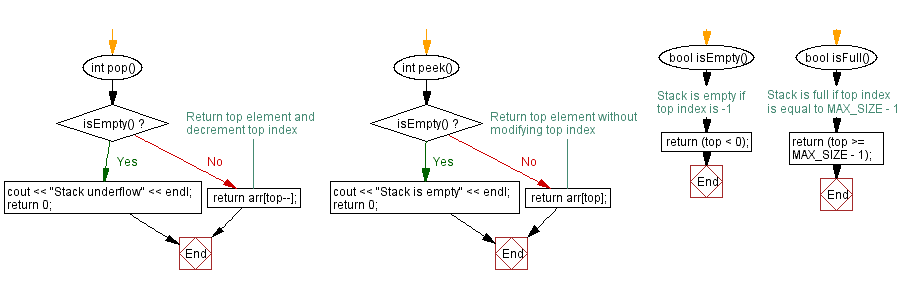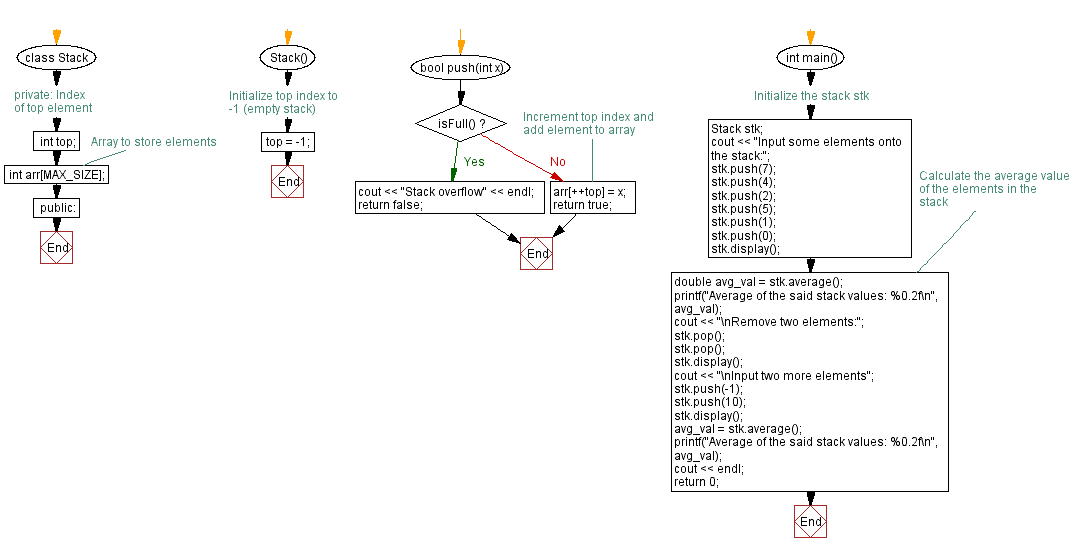﻿ C++ Average value of the stack elements (using an array)

# C++ Stack Exercises: Average value of the stack elements (using an array)

## C++ Stack: Exercise-5 with Solution

Write a C++ program to calculate the average value of the stack (using an array) elements.

Test Data:
Input some elements onto the stack:
Stack elements: 0 1 5 2 4 7
Average of the said stack values: 3.17

Sample Solution:

C++ Code:

``````#include <iostream>

using namespace std;
#define MAX_SIZE 15 // Maximum size of stack

class Stack {
private:
int top; // Index of top element
int arr[MAX_SIZE]; // Array to store elements
public:
Stack() {
top = -1; // Initialize top index to -1 (empty stack)
}

bool push(int x) {
if (isFull()) {
cout << "Stack overflow" << endl;
return false;
}
// Increment top index and add element to array
arr[++top] = x;
return true;
}

int pop() {
if (isEmpty()) {
cout << "Stack underflow" << endl;
return 0;
}
return arr[top--];
}

int peek() {
if (isEmpty()) {
cout << "Stack is empty" << endl;
return 0;
}
return arr[top];
}

bool isEmpty() {
// Stack is empty if top index is -1
return (top < 0);
}

bool isFull() {
// Stack is full if top index is equal to MAX_SIZE - 1
return (top >= MAX_SIZE - 1);
}

void display() {
if (top < 0) {
cout << "Stack is empty" << endl;
return;
}
cout << "\nStack elements: ";
for (int i = top; i >= 0; i--)
cout << arr[i] << " ";
cout << endl;
}

double average() {
if (isEmpty()) {
return 0.0; // Return 0 if the stack is empty
}
int sum = 0;
for (int i = 0; i <= top; i++) {
sum += arr[i]; // Calculate the sum of all elements in the stack
}
return (double) sum / (top + 1); // Return the average value
}
};

int main() {
//Initialize the stack stk
Stack stk;
cout << "Input some elements onto the stack:";
stk.push(7);
stk.push(4);
stk.push(2);
stk.push(5);
stk.push(1);
stk.push(0);
stk.display();
// Calculate the average value of the elements in the stack
double avg_val = stk.average();
printf("Average of the said stack values: %0.2f\n", avg_val);
cout << "\nRemove two elements:";
stk.pop();
stk.pop();
stk.display();
cout << "\nInput two more elements";
stk.push(-1);
stk.push(10);
stk.display();
avg_val = stk.average();
printf("Average of the said stack values: %0.2f\n", avg_val);
cout << endl;
return 0;
}
``````

Sample Output:

```Input some elements onto the stack:
Stack elements: 0 1 5 2 4 7
Average of the said stack values: 3.17

Remove two elements:
Stack elements: 5 2 4 7

Input two more elements
Stack elements: 10 -1 5 2 4 7
Average of the said stack values: 4.50
```

Flowchart:CPP Code Editor: